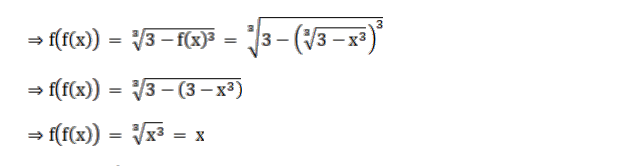# Mark (√) against the correct answer in the following:Question:

Mark (√) against the correct answer in the following:

If $f(x)=\sqrt{3-x^{3}}$ then ( $f$ of) $(x)=?$

A $\mathrm{X}^{1 / 3}$

B. $x$

C. $\left(1-\mathrm{x}^{1 / 3}\right)$

D. None of these

Solution:

$f(x)=\sqrt{3-x^{3}}$